# Labelling Barplot with ggplotAssist(I)

#### 2017-11-13

In this vignette, you will learn how to add labels to bar plots representing counts. Labelling bar plots representing values will be coverd in the next vignette. You can add labels using geom_text() function. If you want to add labels to the proportional bar plots, you have to make some manipulation of your data.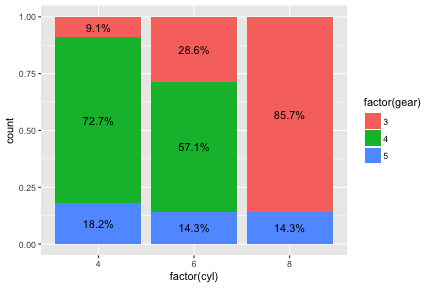# Bar plot representing count

## Stacked bar plot

The default position of the geom_bar() function is stack and the default stat is count. You can make bar plot with geom_bar(stat=“count”). This is identical with stat_count(geom=“bar”).

ggplot(mtcars,aes(x=factor(cyl),fill=factor(gear)))+geom_bar(stat="count")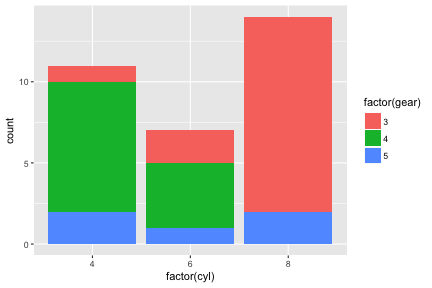ggplot(mtcars,aes(x=factor(cyl),fill=factor(gear)))+stat_count(geom="bar")You can add labels to bar plot using geom_text(). You can add labels represneting counts with the following code. The geom_text() needs x,y and label.

ggplot(mtcars,aes(x=factor(cyl),fill=factor(gear)))+
geom_bar()+
geom_text(aes(label=..count..),stat="count",position=position_stack())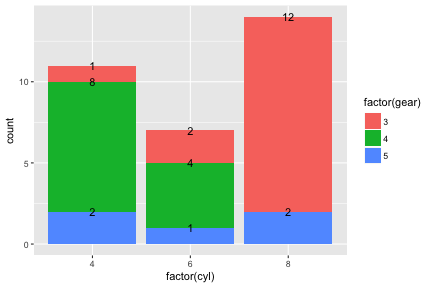If you want to put the label in the middle of each bar, set the vjust argument of position_stack() function to 0.5.

ggplot(mtcars,aes(x=factor(cyl),fill=factor(gear)))+
geom_bar()+
geom_text(aes(label=..count..),stat="count",position=position_stack(0.5))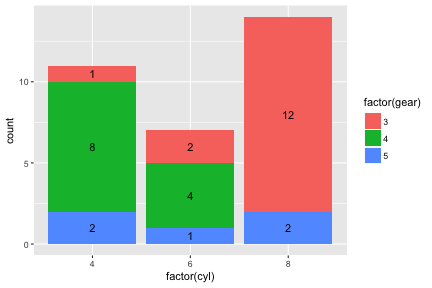## labelling stacked barplot with ggplotAssist

### Mapping the variables

Launch ggplotAssist add-in. Check the data name(1). After select the as factor checkbox(2), select x and factor(cyl) to map the x-axis variable(not shown). Select fill(3) and factor(gear)(arrow) to map the fill variable.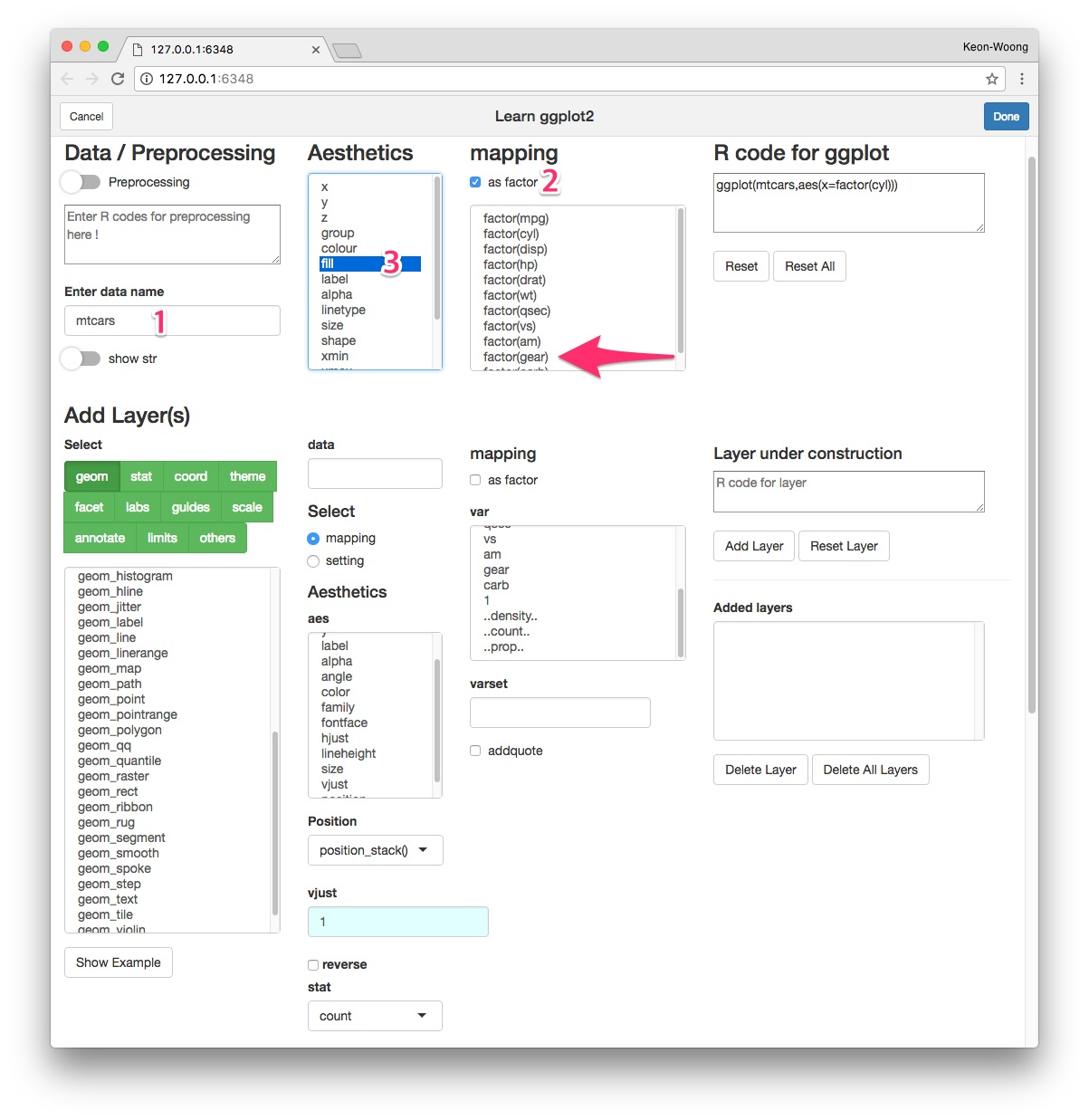Select geom button(4). Select geom_bar(5) among selections. Check the R code for the layer(6) and plot preview in the lower right corner. Press Add Layer button(7).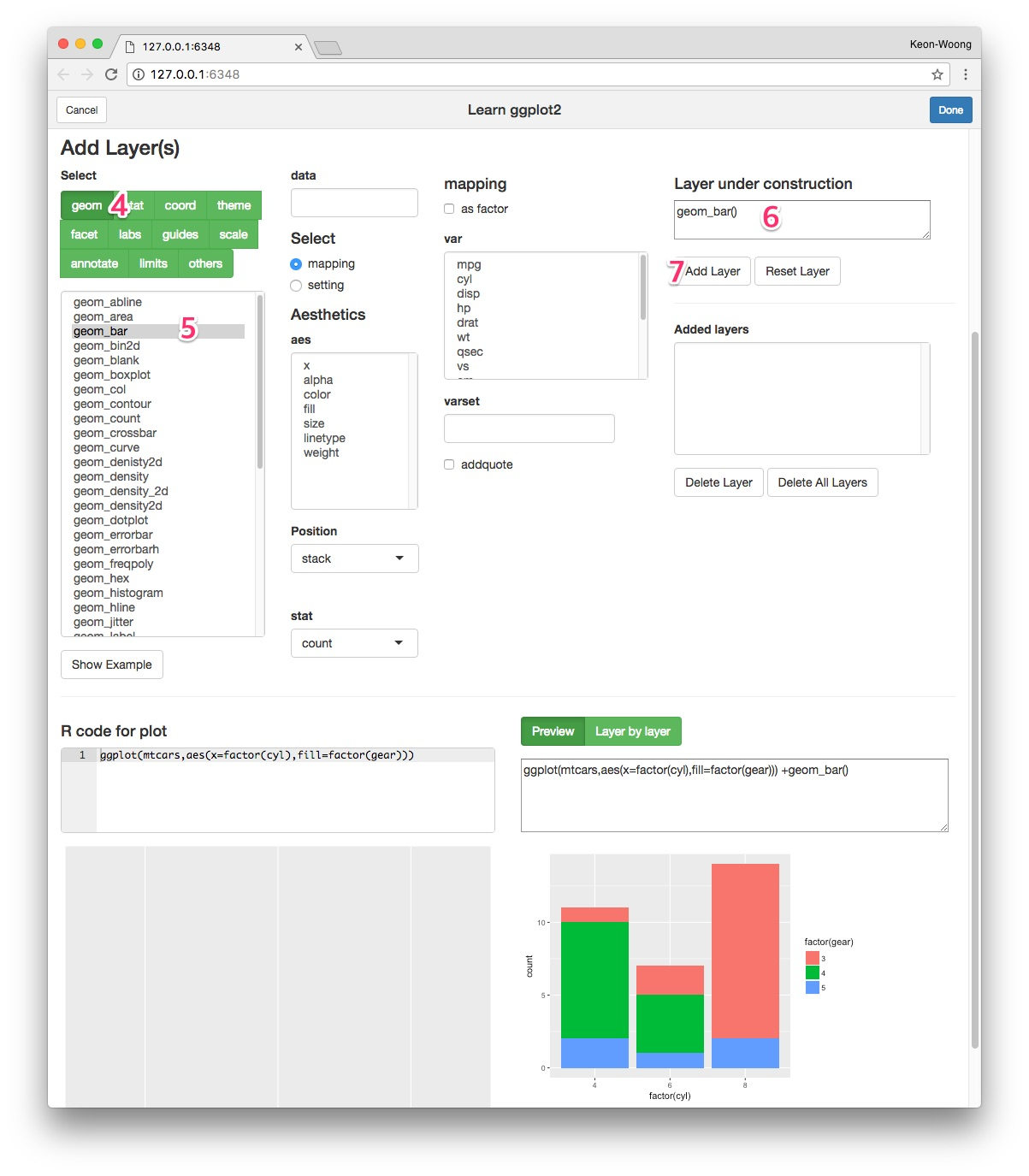### Add geom_text() layer - standard method

Select geom_text(8) among geoms. To map label, select mapping radioButton(9). Select label among aes(10) and select ..count.. among var(11). Select count as stat(12). Select position_stack() as position(13) and set vjust 0.5(14). Check the resultant R code for layer(15) and plot preview at lower right corner. Press Add Layer button(16).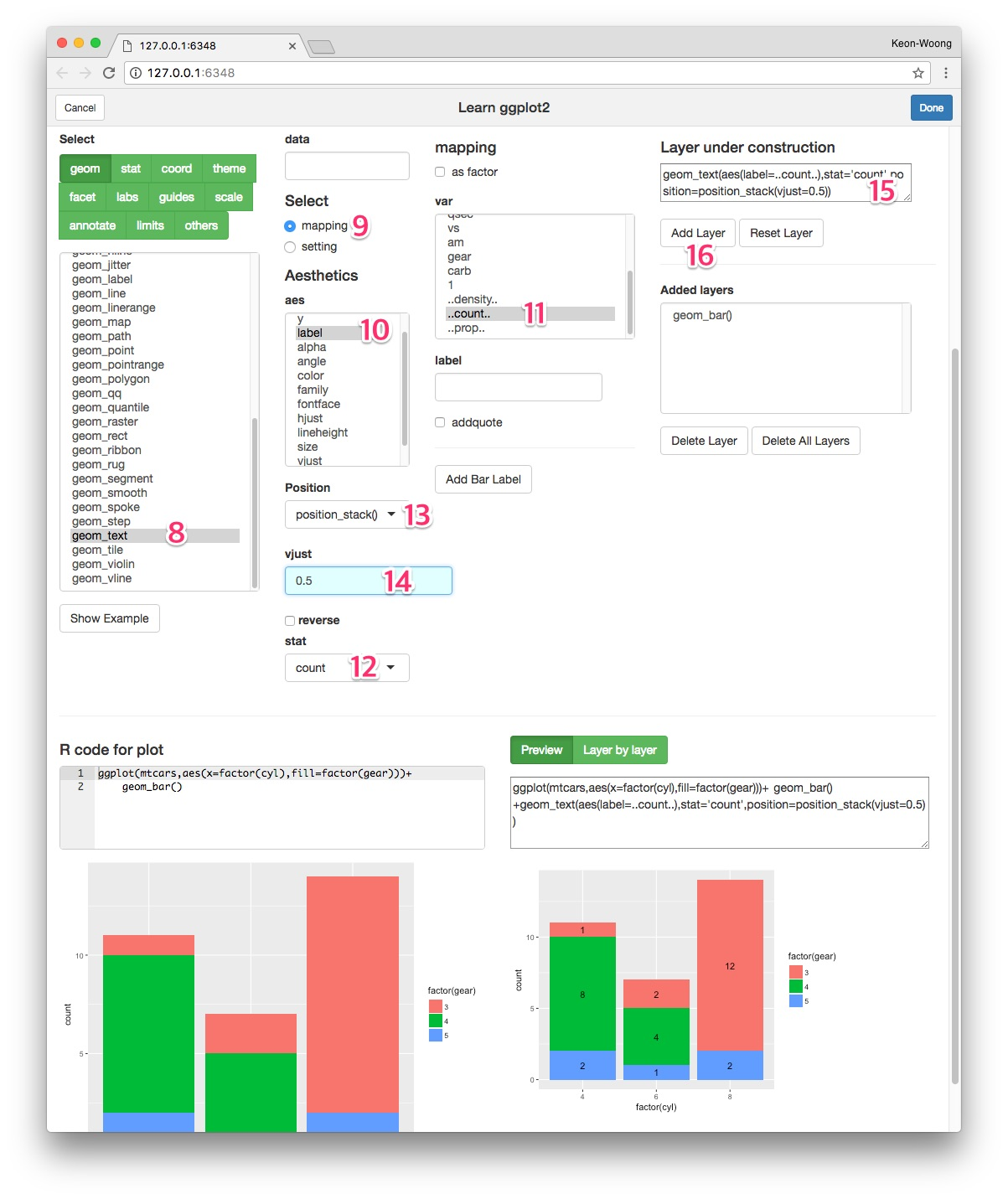### Add geom_text() layer - A shortcut

I have made a shortcut in the ggplotAssist app. After adding the geom_bar() layer, select geom_text(8) among geoms. Press Add Bar Label button(9). The R code for labelling bar plot is made(10). Adjust vjust argument(11).